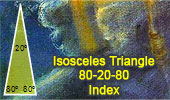# Isosceles Triangle 80-20-80, Theorems and Problems - Table of ContentLangley's Problem Adventitious angles. 20° isosceles triangle with animation.

Geometry Problem 1508.
Understanding Triangle Geometry: Isosceles, Equilateral, Quadrilateral, Angular. Difficulty Level: High School.

Geometry Problem 1389.
Triangle, 40-120-20 degree, Angle, Congruence.

Geometry Problem 1388.
Triangle 40-60-80 degree, Incenter, Congruence.

Problem 1341.
Geometry Problem 1341: Isosceles Triangle, 80-20-80 Degrees, Circumcenter, Angle Bisector.

Geometry Problem 1274.
Isosceles Triangle, 80-20-80 Degrees, Area, Inradius, Circumradius, Angle Bisector, Metric Relations, Measurement.

Geometry Problem 1038.
Isosceles Triangle, Angle, 80, 20, 25, 65 Degrees.

Geometry Problem 691.
Isosceles Triangle, 80-80-20 Degrees, Angles, Congruence, Mind Map, Polya
.

Geometry Problem 688.
Triangle, Angles, 10, 20, 30, 40, 60 Degrees, Measure, Mind Map, Polya
.

Proposed Problem 358.
Isosceles triangle 80-80-20, Circle, Angles, Congruence.

Proposed Problem 274.
Isosceles Triangle, 80-80-20, Angles.

Problem 10.
Triangle, cevian, equal segments, angles, 80-20 degrees
Solution.